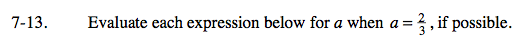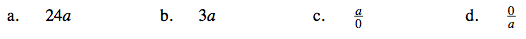### Home > AC > Chapter 7 > Lesson 7.1.2 > Problem7-13

7-13.
1. Evaluate each expression below for a when a =, if possible. Homework Help ✎

1. 24a

2. 3a

3.4.Multiply 24 by 2, then divide by 3.

$\frac{24}{1} \cdot \frac{2}{3}= \frac{48}{3}= 16$

Dividing by 0 = undefined.

Use the same method as in part (a).

0